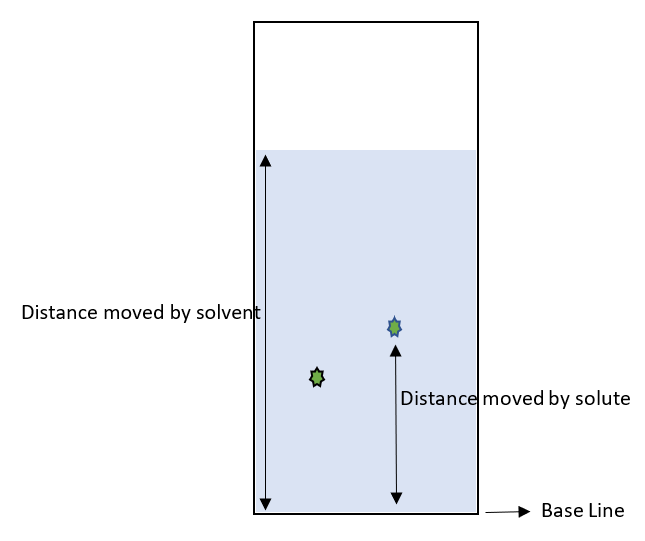# Retention Factor Formula

• Last Updated : 03 Mar, 2022

A solution is a mixture of solute and solvent. In paper chromatography when a solution is placed at the base level, The solute and solvent travel some distance. The components will retain on paper. The amount of retention can be expressed using a factor called Retention Factor.

### Retention Factor Formula

Retention Factor can be defined as the ratio of distance traveled by solute to the distance traveled by solvent. It is denoted by Rf. The formula is given by,

Retention Factor (Rf) =The schematic representation is for the above formula is,These replacement factors of various solutions are used to make comparisons between them with different combinations of solute and solvents. The Rf values vary from one solution to another solution. Interactions of individual components with each other and concentration of the component in the sample will affect the Retention factor value.

### Sample Problems

Question 1: Calculate the retention factor if the solute and solvent moved from 5cm and 10cm respectively from the baseline which is at 0cm.

Solution:

Given,

Distance moved by solute from base line = 5cm

Distance moved by solvent from base line = 10cm

Retention Factor (Rf) == 5/10

= 1/2

= 0.5

Retention Factor for the given solution is 0.5

Question 2: Calculate the retention factor if the solute and solvent moved from 7cm and 20cm respectively from the baseline which is at 0cm.

Solution:

Given,

Distance moved by solute from base line = 7cm

Distance moved by solvent from base line = 20cm

Retention Factor (Rf) == 7/20

= 0.35

Retention Factor for the given solution is 0.35

Question 3: Calculate the retention factors for solute A and B if they moved from 2cm and 5cm from base level and solvent moved to 10cm up from the baseline which is at 0cm.

Solution:

Given,

Distance moved by solute A from base line = 2cm

Distance moved by solute B from base line = 5cm

Distance moved by solvent from base line = 10cm

Retention Factor of A (Rf) == 2/10

= 0.2

Retention Factor of B (Rf) == 5/10

= 0.5

Retention Factor of solute A, B are 0.2, 0.5

Question 4: Calculate the retention factors for solute A and B if they moved from 10cm and 20cm from base level and solvent moved to 60cm up from the baseline which is at 0cm.

Solution:

Given,

Distance moved by solute A from base line = 10cm

Distance moved by solute B from base line = 20cm

Distance moved by solvent from base line = 60cm

Retention Factor of A (Rf) == 10/60

= 0.16

Retention Factor of B (Rf) == 20/60

= 0.33

Retention Factor of solute A, B are 0.16, 0.33

Question 5: Calculate the retention factor if the solute is moved to 23% above the base level and solvent moved up to 50%.

Solution:

Given,

Distance moved by solute from base line = 23% = 23/100 = 0.23 units

Distance moved by solvent from base line = 50% = 50/100 = 0.5 units

Retention Factor (Rf) == 0.23/0.5

= 0.46

Retention Factor for the given solution is 0.46

My Personal Notes arrow_drop_up